# On planarity of compressed zero-divisor graphs associated to commutative rings

### Abstractcreative_2020_29_2_131_136_abstract

Issue no:

The equivalence class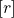of an element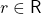is the set of zero-divisors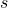such that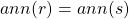, that is,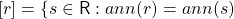. The compressed zero-divisor graph, denoted by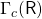, is the compression of a zero-divisor graph, in which the vertex set is the set of all equivalence classes of nonzero zero-divisors of a ring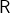, that is, the vertex set ofis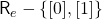, where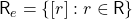and two distinct equivalence classesand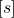are adjacent if and only if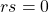. In this article, we investigate the planarity offor some finite local rings of order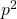,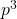and determine the planarity of compressed zero-divisor graph of some local rings of order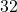, whose zero-divisor graph is nonplanar. Further, we determine values ofand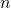for which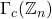and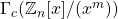are planar.